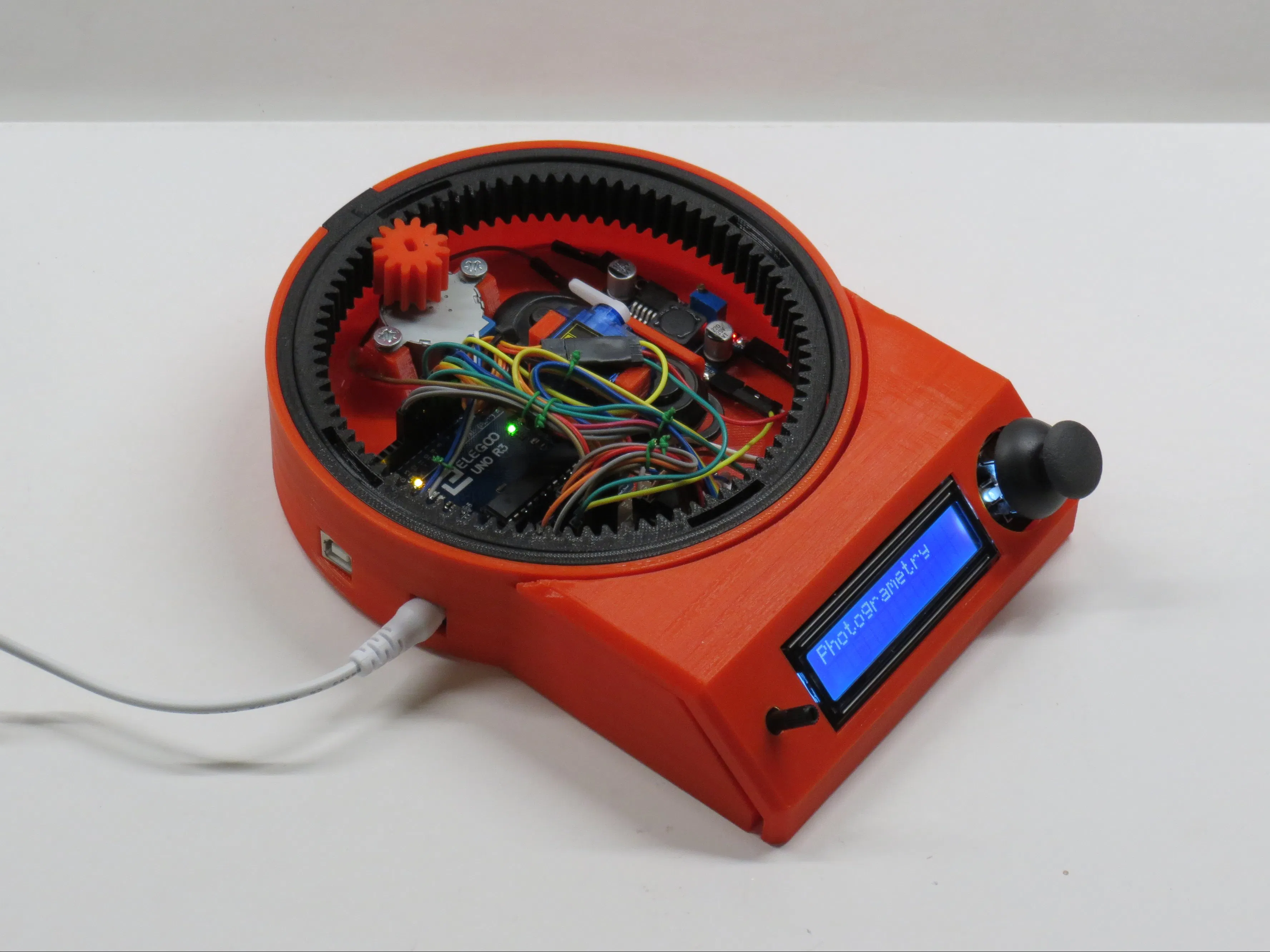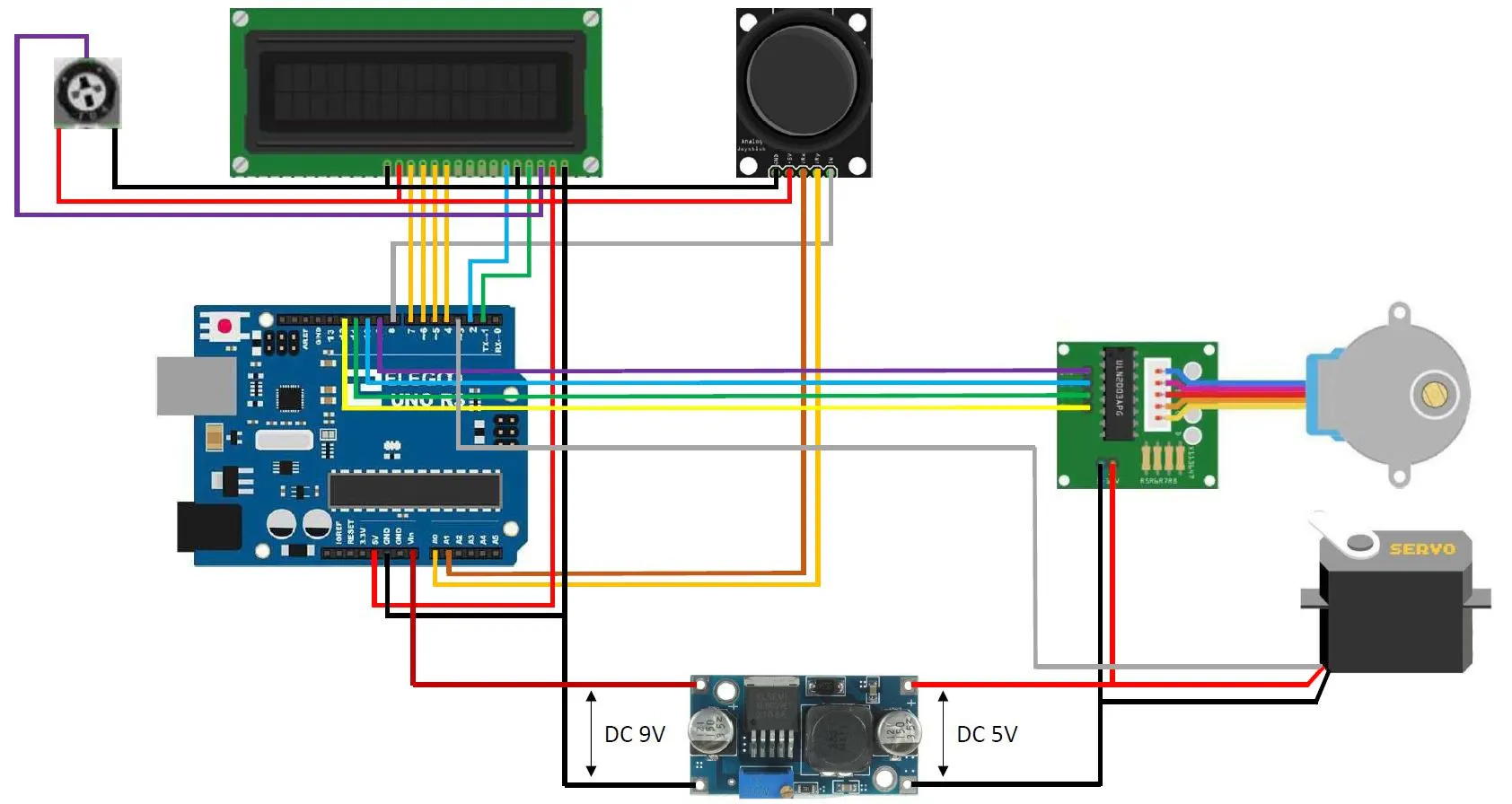Published © CC BY-NC-ND

# Arduino-Controlled Photogrammetry 3D Scanner

A completely automated way to take photos and convert them into a 3D object.

IntermediateFull instructions providedOver 1 day3,414## Things used in this project

### Hardware components

 9g Servo motor
×1
 1602 blue LCD-screen
×1
 Arduino uno
×1
 Double sided prototype board 4cmX6cm type: 107822J2H
×1
 DC-DC step down regulator
×1
 Joystick
×1
 stepper motor and driver
×1
 Bluetooth remote
×1

### Hand tools and fabrication machines

 3D-printer A 3D-printer with a very nice quality/price ratio.

Read more

## Schematics

### wiring diagram

wiring diagram for the turntable## Code

### Arduino program

C/C++
Arduino program for the turntable. It works but keep in mind there is room for improvement.
```#include <LiquidCrystal.h>
#include <Stepper.h>
#include <Servo.h>

LiquidCrystal lcd(1, 2, 4, 5, 6, 7);  // Use these pins for the 1602 lcd

const int SW_pin = 8; // digital pin connected to switch output
const int X_pin = A0; // analog pin connected to X output
const int Y_pin = A1; // analog pin connected to Y output

int MenuNr = 0;   // Menu number
int PhotoNr = 2;  // The amount of photos that have to be taken
bool Flag1 = 0;   // This flag is only active during 1 program cycle (prevents constantly adding/subtracting 1 to the menu number when the joystick is pushed to the side)
bool Flag2 = 0;   // This flag is only active during 1 program cycle (prevents constantly adding/subtracting 2 to the photo number when the joystick is pushed up or down)
bool Flag3 = 0;   // This flag is only active during 1 program cycle (prevents constantly adding/subtracting 1 to the RPM when the joystick is pushed up or down)
bool Flag4 = 0;   // This flag is only active during 1 program cycle (prevents constantly adding/subtracting 1 to the turn number when the joystick is pushed to the side)
bool Flag5 = 0;   // This flag is only active during 1 program cycle (prevents constantly adding/subtracting 1 to the RPM when the joystick is pushed up or down)
bool Flag6 = 0;   // This flag is only active during 1 program cycle to clear the lcd
int SwMenu = 0;   // Switch menu (Sub menu's in the main menu's)
bool BtnFlag = 0; // This flag is only active during 1 program cycle (prevents constantly adding of 1 to SwMenu when button is pressed)

const int stepsPerRevolution = 2048;  // change this to fit the number of steps per revolution
int FullRev = 14336;                  // 1 full revolution of the big gear -> Small-Big gear ratio is 7:1
int rolePerMinute = 15;               // Adjustable range of 28BYJ-48 stepper is 0~17 rpm
int PhotoTaken = 0;                   // Amount of photo's that have been taken
int StepPerPhoto;                     // Amount of steps per photo (calculated -> see MenuNr 0/SwMenu 2)
int TurnNr = 1;                       // Amount of turns
int CurrentTurn = 0;                  // Stores current turn number
int Steps = 0;                        // Amount of individual steps the stepper motor has to turn

Stepper myStepper(stepsPerRevolution, 9, 11, 10, 12);  // Use these pins for the stepper motor

Servo Servo1;  // Define Servo1 as a servo

void setup() {
lcd.begin(16, 2);                   //Lcd setup

pinMode(SW_pin, INPUT);             //Set pushbutton as input
digitalWrite(SW_pin, HIGH);         //Set SW_pin High

myStepper.setSpeed(rolePerMinute);  //Set RPM of steppermotor

Servo1.attach(3);                   //Attach servo to pin 3
Servo1.write(90);                   //Move servo to mid possition

lcd.setCursor(4, 0);                //Startup screen start
lcd.print("Welcome!");              //      """""      //
lcd.setCursor(1, 1);                //      """""      //
lcd.print("Software: V1.6");        //      """""      //
delay(3000);                        //      """""      //
lcd.clear();                        //      """""      //
lcd.setCursor(0, 0);                //      """""      //
lcd.print("Designed by");           //      """""      //
lcd.setCursor(0, 1);                //      """""      //
lcd.print("Brian Brocken");         //      """""      //
delay(2000);                        //      """""      //
lcd.clear();                        //Startup screen end
}

void loop() {

int XValue = analogRead(X_pin);     // Read the analog value from The X-axis from the joystick
int YValue = analogRead(Y_pin);     // Read the analog value from The Y-axis from the joystick
int SwValue = digitalRead(SW_pin);  // Read the digital value from The Button from the joystick

if (MenuNr < 0){  //This sets the min number of menu's
MenuNr = 0;
}
else if ( MenuNr > 2){  //This sets the max numbers of menu's
MenuNr = 2;
}

if (XValue < 400 && Flag1 == 0 && SwMenu == 0){  //if the joystick is pushed to the right side and flag1 is 0 then 1 will be added to the menu number (purpose of the flag -> see comment Flags above)
MenuNr = MenuNr + 1;
Flag1 = 1;
lcd.clear();
}

if (XValue > 600 && Flag1 == 0 && SwMenu == 0){  //if the joystick is pushed to the left side and flag1 is 0 then 1 will be subtracted from the menu number (purpose of the flag -> see comment Flags above)
MenuNr = MenuNr - 1;
Flag1 = 1;
lcd.clear();
}

if (XValue > 399 && XValue < 599 && Flag1 == 1){  //if joystick is at neutral possition, flag1 is set to 0 (purpose of the flag -> see comment Flags above)
Flag1 = 0;
}

if (SwValue == 0 && BtnFlag == 0){  //if the button is pressed and the flag is 0 -> add 1 to menu
SwMenu = SwMenu + 1;
BtnFlag = 1;
lcd.clear();
}

if (SwValue == 1 && BtnFlag == 1){  //if the button is not pressed and the flag is 0 -> Reset the flag (purpose of the flag -> see comment Flags above)
BtnFlag = 0;
}

//***********************************************Menu0***********************************************//

if (MenuNr == 0){  //Menu0 program

if (SwMenu == 0){ //Menu 0 selected
lcd.setCursor(0, 0);
lcd.print("Photogrametry");
}

if (SwMenu == 1){ //entered menu 0
lcd.setCursor(0, 0);
lcd.print("Nr of photo's");
lcd.setCursor(7, 1);
lcd.print(PhotoNr);

if (YValue < 400 && Flag2 == 0){ //joystick up -> Add 2 to number of photo's
PhotoNr = PhotoNr + 2;
Flag2 = 1;
lcd.clear();
}
if (YValue > 600 && Flag2 == 0){ //joystick down -> Subtract 2 from number of photo's
PhotoNr = PhotoNr - 2;
Flag2 = 1;
lcd.clear();
}
if (YValue > 399 && YValue < 599 && Flag2 == 1){  //if the Y-axis is back at it's neutral possition -> Flag3 = 0 -> Prevents constant adding or subtracting of 2
Flag2 = 0;
}

if (PhotoNr < 2){    //Min allowable Nr of photo's
PhotoNr = 2;
}
if (PhotoNr > 200){  //Max allowable Nr of photo's
PhotoNr = 200;
}
}

if (SwMenu == 2){ //Program started

lcd.setCursor(0, 0);
lcd.print("Program started");
lcd.setCursor(0, 1);
lcd.print("Photo Nr: ");
lcd.print(PhotoTaken);

StepPerPhoto = FullRev / PhotoNr;  //Calculate amount of steps per photo

if (PhotoTaken < PhotoNr){
myStepper.setSpeed(rolePerMinute);  //Set motor speed
myStepper.step(StepPerPhoto);       //move the calculated amount of steps
PhotoTaken = PhotoTaken + 1;        //Add 1 to photos taken
lcd.setCursor(0, 1);
lcd.print("Photo Nr: ");            //Taking photo's
lcd.print(PhotoTaken);
Servo1.write(30);
delay(300);
Servo1.write(90);
delay(1000);
}

if (PhotoTaken == PhotoNr){  //If the amount of photos taken is equal to the amount of photos that have to be taken -> Program finished
lcd.setCursor(0, 0);
lcd.print("Program finished");
delay(3000);
lcd.clear();  //Rest parameters
PhotoTaken = 0;
PhotoNr = 2;
SwMenu = 0;
Steps = 0;
}
}
}

//***********************************************Menu1***********************************************//

if (MenuNr == 1){  //Menu1 program

if (SwMenu == 0){  //Menu1 selected
lcd.setCursor(0, 0);
lcd.print("Cinematic");
}

if (SwMenu == 1){  //Entered menu1 - sub menu1
lcd.setCursor(0, 0);
lcd.print("motor speed");
lcd.setCursor(7, 1);
lcd.print(rolePerMinute);

if (YValue < 400 && Flag3 == 0){ // joystick up -> Add 1 RPM
rolePerMinute = rolePerMinute + 1;
Flag3 = 1;
lcd.clear();
}
if (YValue > 600 && Flag3 == 0){ // joystick down -> Subtract 1 RPM
rolePerMinute = rolePerMinute - 1;
Flag3 = 1;
lcd.clear();
}
if (YValue > 399 && YValue < 599 && Flag3 == 1){  //if the Y-axis is back at it's neutral possition -> Flag3 = 0 -> Prevents constant adding or subtracting of 1
Flag3 = 0;
}

if (rolePerMinute < 1){  //Min allowable RPM
rolePerMinute = 1;
}
if (rolePerMinute > 17){  //Max allowable RPM
rolePerMinute = 17;
}
}

if (SwMenu == 2){  //Entered menu1 - sub menu2
lcd.setCursor(0, 0);
lcd.print("Nr of turns");
lcd.setCursor(7, 1);
lcd.print(TurnNr);

if (YValue < 400 && Flag4 == 0){ // joystick up -> Add 1 turn
TurnNr = TurnNr + 1;
Flag4 = 1;
lcd.clear();
}
if (YValue > 600 && Flag4 == 0){ // joystick down -> Subtract 1 turn
TurnNr = TurnNr - 1;
Flag4 = 1;
lcd.clear();
}
if (YValue > 399 && YValue < 599 && Flag4 == 1){  //if the Y-axis is back at it's neutral possition -> Flag3 = 0 -> Prevents constant adding or subtracting of 1
Flag4 = 0;
}

if (TurnNr < 1){  //Min allowable amount of turns
TurnNr = 1;
}
if (TurnNr > 200){  //Max allowable amount of turns
TurnNr = 200;
}
}

if (SwMenu == 3){  //Program started
lcd.setCursor(0, 0);
lcd.print("Program started");
lcd.setCursor(0, 1);
lcd.print("Turns done: ");
lcd.print(CurrentTurn);

if (CurrentTurn < TurnNr){
myStepper.setSpeed(rolePerMinute);
myStepper.step(FullRev);
CurrentTurn = CurrentTurn + 1;
lcd.setCursor(0, 1);
lcd.print("Turns done: ");
lcd.print(CurrentTurn);
}

if (CurrentTurn == TurnNr){  //If the current turn is equal to the amount of thurns that need to be turned -> program finished
lcd.setCursor(0, 0);
lcd.print("Program finished");
delay(3000);
lcd.clear();  //Reset
CurrentTurn = 0;
PhotoNr = 1;
rolePerMinute = 15;
SwMenu = 0;
Steps = 0;
}

}
}

//***********************************************Menu2***********************************************//

if (MenuNr == 2){  //Menu2 selected

if (SwMenu == 0){
lcd.setCursor(0, 0);
lcd.print("Manual control");
}

if (SwMenu == 1){  //Entered menu2
lcd.setCursor(0, 0);
lcd.print("motor speed");
lcd.setCursor(7, 1);
lcd.print(rolePerMinute);

if (YValue < 400 && Flag5 == 0){ // joystick up -> Add 1 RPM
rolePerMinute = rolePerMinute + 1;
Flag5 = 1;
lcd.clear();
}
if (YValue > 600 && Flag5 == 0){ // joystick down -> Subtract 1 RPM
rolePerMinute = rolePerMinute - 1;
Flag5 = 1;
lcd.clear();
}
if (YValue > 399 && YValue < 599 && Flag5 == 1){  //if the Y-axis is back at it's neutral possition -> Flag3 = 0 -> Prevents constant adding or subtracting of 1
Flag5 = 0;
}

if (rolePerMinute < 1){  //Min allowable RPM
rolePerMinute = 1;
}
if (rolePerMinute > 17){  //Max allowable RPM
rolePerMinute = 17;
}

if (XValue < 400 ){  //if the joystick is pushed to the right side and the neutral flag is 0 then 1 will be added to the menu number (purpose of the flag -> see comment Flag1 above)
Steps = Steps + 1;
myStepper.setSpeed(rolePerMinute);
myStepper.step(Steps);
lcd.setCursor(14, 1);
lcd.print("->");
Flag6 = 1;
}

if (XValue > 600 ){  //if the joystick is pushed to the left side and the neutral flag is 0 then 1 will be subtracted from the menu number (purpose of the flag -> see comment Flag1 above)
Steps = Steps + 1;
myStepper.setSpeed(rolePerMinute);
myStepper.step(-Steps);
lcd.setCursor(0, 1);
lcd.print("<-");
Flag6 = 1;
}

if (XValue > 399 && XValue < 599){  //if the Y-axis is back at it's neutral possition -> Flag3 = 0 -> Prevents constant adding or subtracting of 1
Steps = 0;
if (Flag6 == 1){
lcd.clear();
Flag6 = 0;  //This flag is only active during 1 program cycle to clear the lcd
}
}
}

if (SwMenu == 2){  //Leave menu 2 when button is pressed
lcd.clear();
CurrentTurn = 0;
PhotoNr = 1;
rolePerMinute = 15;
SwMenu = 0;
Steps = 0;
}
}
}
```

## Credits

### Bribro12

19 projects • 13 followers
I'm a maker. I design and create my own projects and build them from scratch. My favorite tools are my 3D-printer and laser cutter